### Home > CALC > Chapter 8 > Lesson 8.1.4 > Problem8-44

8-44.
1.The Pi hotel is in the town of Acelerton. The hotel features a grand fireworks display every 4th of July. After launching the fireworks from the top of the building, the projectile reaches its maximum height where it explodes, amazing the crowds on the ground. The function that models the height of the shell (in feet) at any time t (in seconds) is given by the function:
s(t) = −16t2 + 120t + 136 Homework Help ✎

1. How tall is the hotel?

2. What is the initial velocity of the projectile?

3. In order to determine the length of the fuse, the organizers need to know when the projectile will be at its maximum height. At what time should the firework explode?

4. How high will the explosion occur?

5. Oh no! The timing device failed! The shell is falling towards the ground. If the shell is traveling faster than 150 ft/sec it will explode on contact with the ground. Will the shell explode?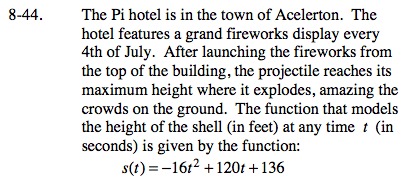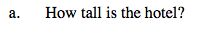Since the distance function tracks the height of the shell, and the shell was launched from the top of the hotel,where was the shell at t = 0?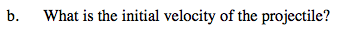You could take the derivative and evaluate at t = 0.
Or you could deconstruct the position function:

$s(t)=\frac{a}{2}t^{2}+v_{0}t+s_{0}.$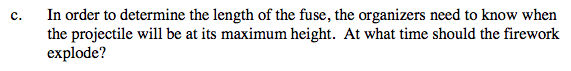Notice that the distance function is a parabola. Use Algebra or Calculus to find the x-value of the vertex of this parabola.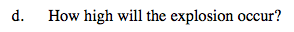Recall that a local maximum (or local minimum) is a y-value, not an x-value. After all, max and min mean highest and lowest, which are vertical measurements (y-axis measurements).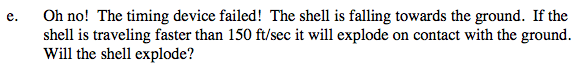Given that s(0) ≠ 0, is it reasonable to assume that the ground is located at s(t) = 0?